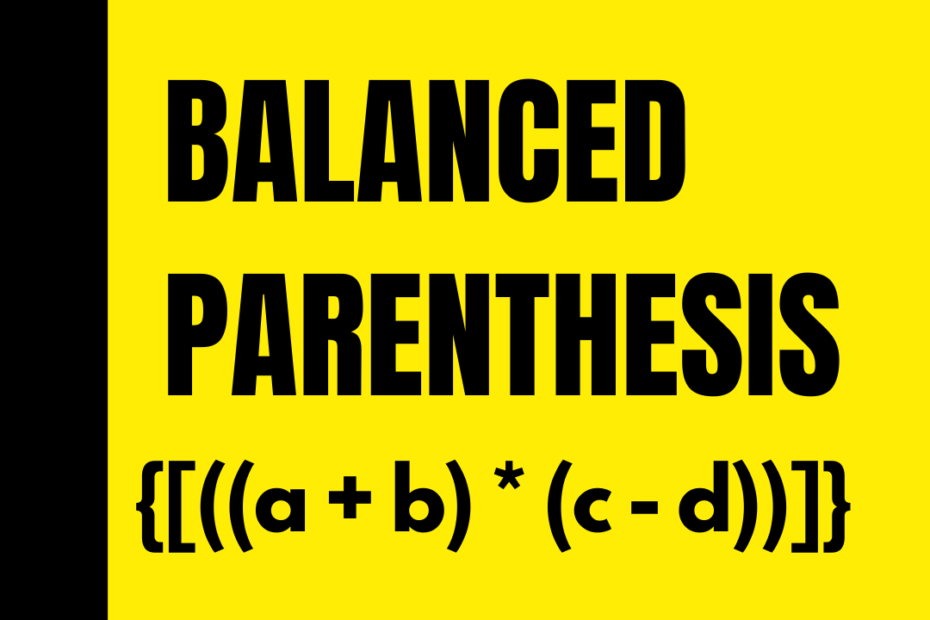# Balanced Parenthesis Program

• DSA

We have an expression that contains brackets and alphanumeric characters, and we need to check whether the parenthesis are balanced or not. Balanced Parenthesis means an equal number of opening and closing brackets.

## Example

``````Input: {(a+b)*(a-b)}
Output: Balanced

Input: {{(x+y)}
Output: Not Balanced``````

## Solution Approach – Using Stack

Why Stack Data Structure?

Here, our priority is to find if there is are an equal number of closing brackets for every opening bracket. If we use a stack, we can directly push() all open brackets into it and pop() them for every closing bracket encountered.

Conditions to follow

• Ignore all alphanumeric and other symbols
• Consider all kinds of brackets/parenthesis[square, curly]

I wrote the program with clear comments and accurate function names have a look at it below.

## Code for Balanced Parenthesis

``````#include <iostream>
using namespace std;

struct Node
{
char data;
struct Node *next;
} *top = NULL; // 'top' points to the Node at the top of the stack

// Time Complexity: O(1)
void push(char x)
{
struct Node *temp;
temp = (struct Node *)malloc(sizeof(struct Node));

if (temp == NULL) // if the heap memory is full
printf("Stack DS is full. No space for new Node.\n");
else
{
temp->data = x;
temp->next = top;
top = temp; // 'temp' node is now the 'top' node
}
}

// Time Complexity: O(1)
char pop()
{
struct Node *temp;
char x = -1;

if (top == NULL)
printf("Stack DS is empty.\n");
else
{
temp = top;
top = top->next;
x = temp->data;
free(temp);
}
return x;
}

int isEmpty()
{
}

char stackTop()
{
if (!isEmpty())
return '0';
}

// Balanced Parenthesis Checker Function
int isBalanced(string exp)
{
int i;
for (i = 0; exp[i] != '\0'; i++)
{

if (exp[i] == '(' || exp[i] == '{' || exp[i] == '{')
push(exp[i]);
else if (exp[i] == ')' || exp[i] == '}' || exp[i] == '}')
{
// edge case where there are more ) than ( in the exp
if (isEmpty())
return 0;
if (stackTop() == '(' && exp[i] == ')')
pop();
else if (stackTop() == '{' && exp[i] == '}')
pop();
else if (stackTop() == '[' && exp[i] == ']')
pop();
else
return 0;
}
}
return isEmpty() ? 1 : 0;
}

int main()
{
string exp = "{[((a + b) * (c - d))]}";
if (isBalanced(exp))
cout<<"The Parenthesis in the Expression are Balanced";
else
cout<<"The Parenthesis in the Expression are Not Balanced";
return 0;
}``````

## Conclusion

This post [Check for Balanced Parenthesis] was a part of my #30DaysChallenge to write a blog post every day on what I learn, Happy Learning~ Abhiram Reddy.